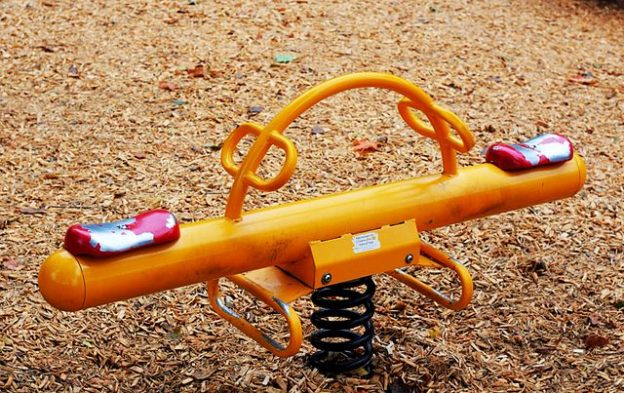Are you more of a visual learner? Check out our online video lectures and start your physics course now for free!Image: “A seesaw or teeter-totter in a children’s playground in Ottawa, Ontario, 2010.” By Photo by M. Rehemtulla. License: CC BY 2.0

## Definition of Torque and Equilibrium

Equilibrium refers to equal forces, implying a state of balance. If an object has forces acting on it, the object may move. However, if the object is in equilibrium, then the object will not move since all the forces balance each other.

One way to look at the motion of an object is to separate the forces acting on the object into horizontal and vertical forces. In equilibrium, the horizontal forces will balance themselves, and the vertical forces will balance themselves. Thus, the object will not move in either the horizontal or vertical axes.

Torque causes an object to rotate about an axis. It depends on the distance between the axis and the point where the force acts. Force multiplied by force arm equals load multiplied by load arm. Torque can be described by the following equation:

M = r F

M ⇒ Torque (N m)

Example: Muscles work on joints, which from a physical pivot point with their axes. When force is exerted upon them, they create a torque.

τ = r F sinΘ

• τ = N m
• Parallel: τ = 0
Since sin 0 = 0
and sin 180 = 0

τ = r F sinΘ

• τ = N m
• Parallel: τ = 0
• Perpendicular: τ = r F

τ = r F sinΘ

• τ = N m
• Parallel: τ = 0
• Perpendicular: τ = r F

τ = F (r sinΘ)
τ = F I

Learn. Apply. Retain.
Your path to achieve medical excellence.
Study for medical school and boards with Lecturio.